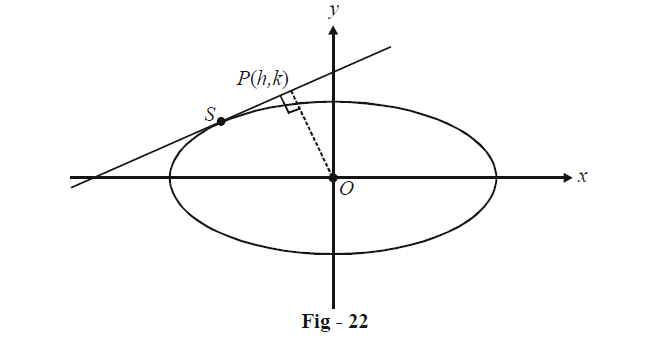# Examples On Tangents To Ellipses Set-2

Go back to  'Ellipse'

Example - 18

Prove that the product of the perpendiculars from the foci upon any tangent to the ellipse \begin{align}\frac{{{x^2}}}{{{a^2}}} + \frac{{{y^2}}}{{{b^2}}} = 1\;is\;{b^2}.\end{align}

Solution: We can assume an arbitrary tangent to this ellipse to be

$y = mx + \sqrt {{a^2}{m^2} + {b^2}}\quad\quad\quad ...\left( 1 \right)$

The perpendicular distances of the two foci, $${F_1}(ae,\,0)\;\text{and}\;{F_2}( - ae,\,0)$$ from the line given by (1) are

\begin{align}&{d_1} = \frac{{mae + \sqrt {{a^2}{m^2} + {b^2}} }}{{\sqrt {1 + {m^2}} }}\\&{d_2} = \frac{{ - mae + \sqrt {{a^2}{m^2} + {b^2}} }}{{\sqrt {1 + {m^2}} }}\end{align}We thus have,

\begin{align}&{d_1}{d_2} = \frac{{{a^2}{m^2} + {b^2} - {a^2}{m^2}{e^2}}}{{1 + {m^2}}}\\&\qquad{\rm{ }} = \frac{{{a^2}{m^2}(1 - {e^2}) + {b^2}}}{{1 + {m^2}}}\\&\qquad{\rm{ }} = \frac{{{m^2}{b^2} + {b^2}}}{{1 + {m^2}}}\\&\qquad{\rm{ }} = {b^2}\end{align}

Example – 19

Find the locus of the foot of the perpendicular drawn from the origin upon any tangent to the ellipse \begin{align}\frac{{{x^2}}}{{{a^2}}} + \frac{{{y^2}}}{{{b^2}}} = 1.\end{align}

Solution: We can assume an arbitrary tangent to this ellipse as

$y = mx + \sqrt {{a^2}{m^2} + {b^2}}$

Let the foot of perpendicular from the origin upon this tangent be P(h, k):Thus,

$k = mh + \sqrt {{a^2}{m^2} + {b^2}} ...\left( 1 \right)$

Also, $$OP \bot SP$$ (the tangent)

\begin{align}&\Rightarrow\quad \frac{k}{h} \times m = - 1\\& \Rightarrow \quad m = - \frac{h}{k}\qquad\qquad...\left( 2 \right)\end{align}

Using (2) in (1), we obtain a relation between h and k as

${({h^2} + {k^2})^2} = {a^2}{h^2} + {b^2}{k^2}$

Thus, the required locus of P is

${({x^2} + {y^2})^2} = {a^2}{x^2} + {b^2}{y^2}$